# Rajasthan Board Question Paper for Class 10 Maths 2016 In PDF

## RBSE 10th Maths Question Papers 2016 With Solutions

Rajasthan Board 10th maths 2016 question paper with solutions In PDF can be downloaded for Free from this Article. Along with the solutions, Students can also find the RBSE 10th maths question paper 2016, which addresses the issue of time management and helps the students allocate time for each question.

Visit and download all the previous year RBSE question papers curated by BYJU’S. These question papers will help the students to know the exact difficulty level of the question papers. Solving 2016 Maths question papers for Class 10 enables a thorough understanding of each and every concept present in the textbook.

### QUESTION PAPER CODE S–09–Mathematics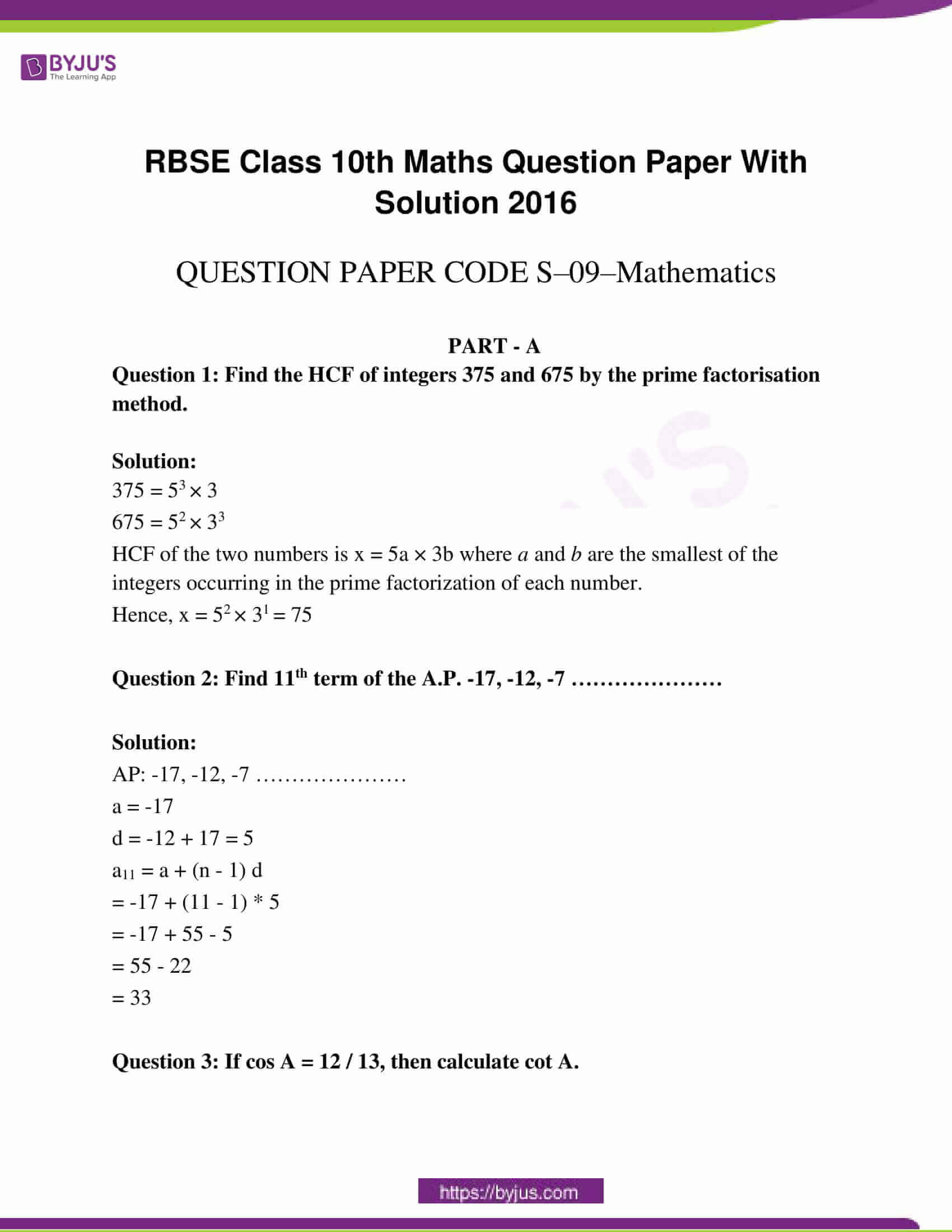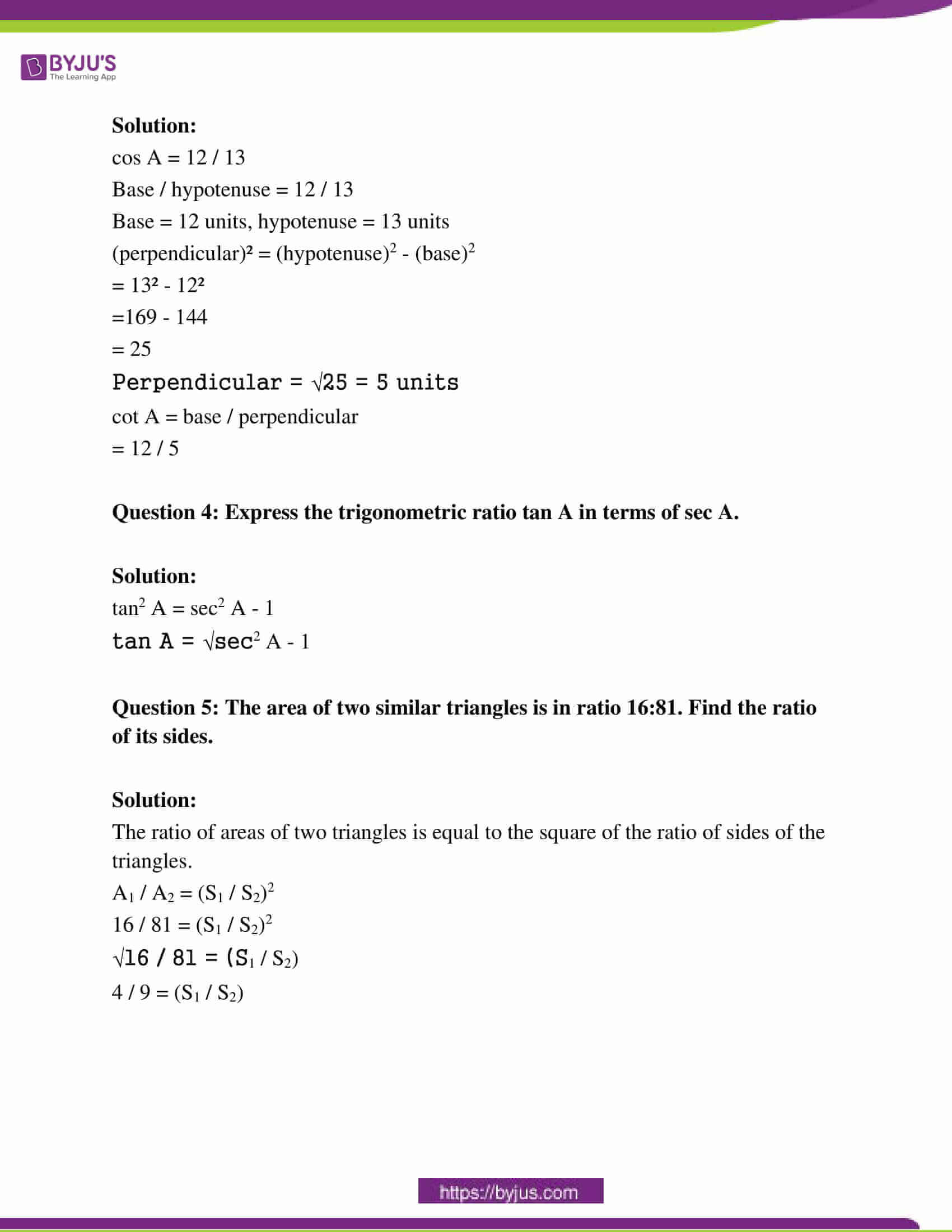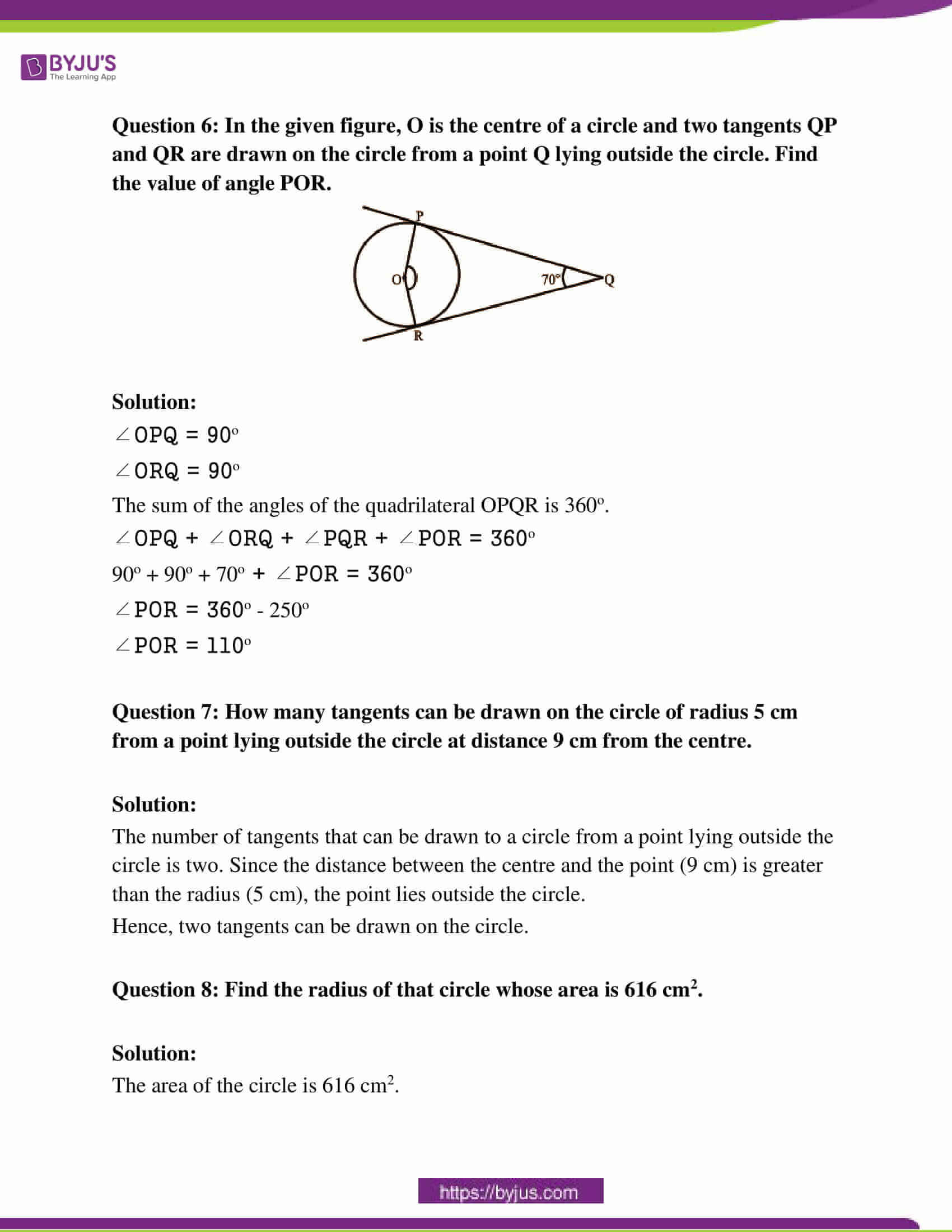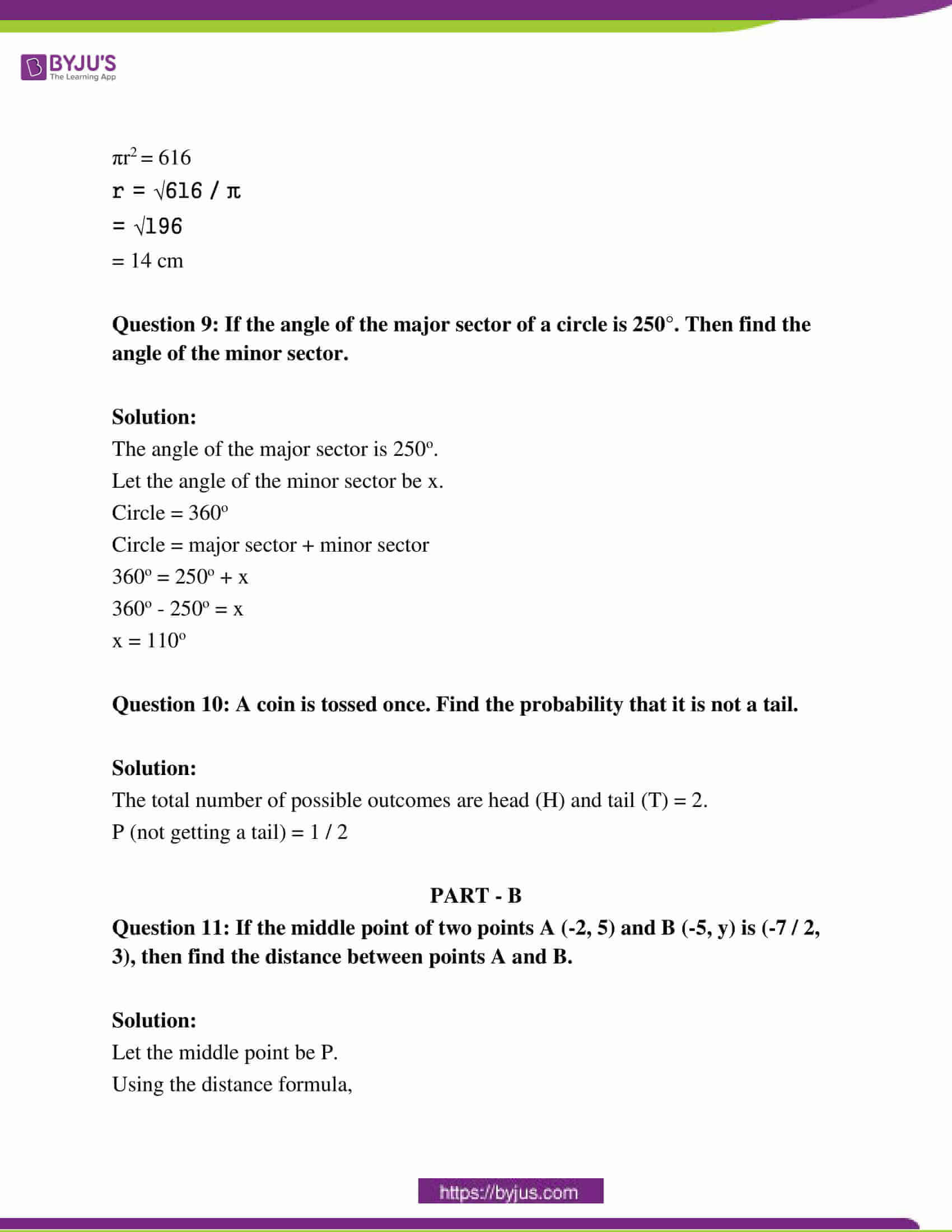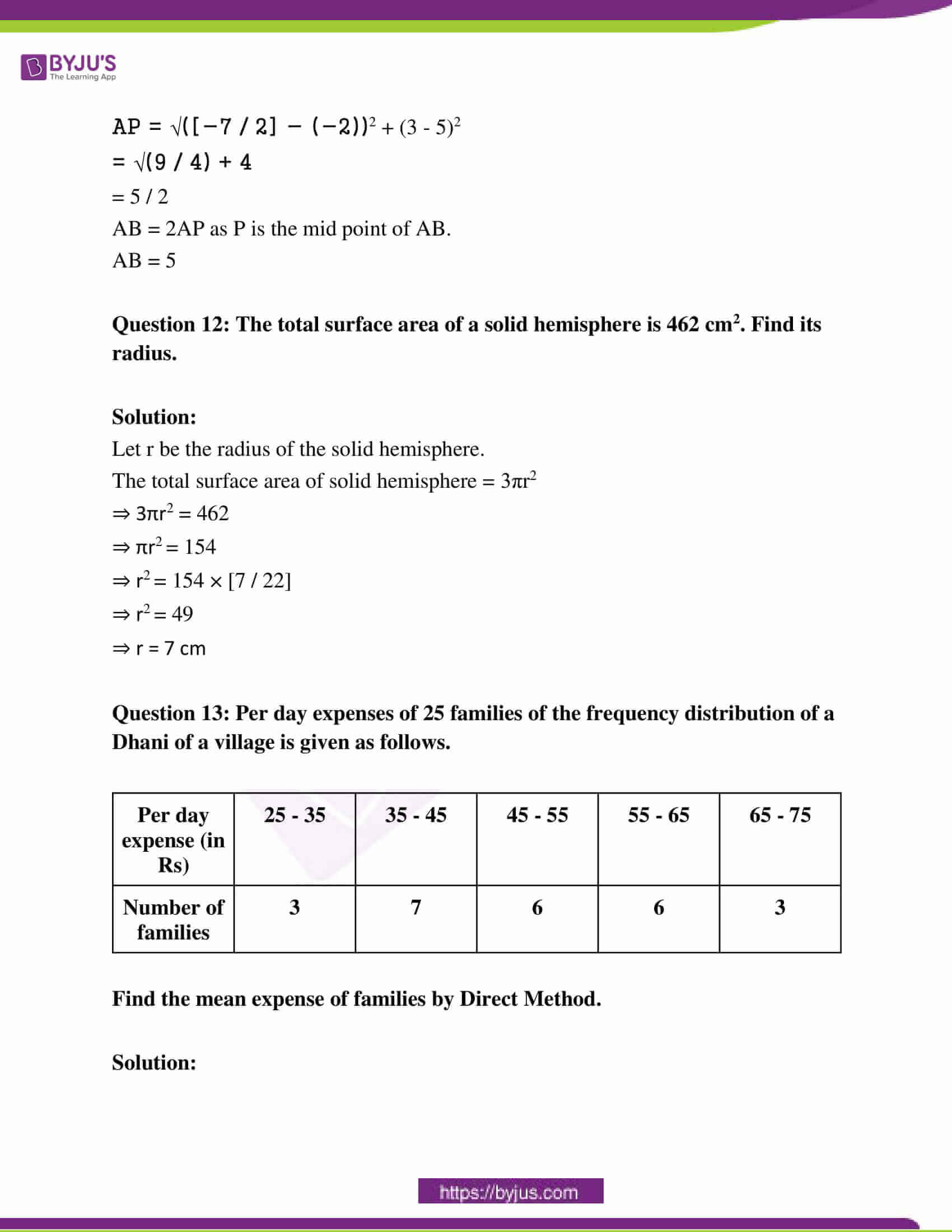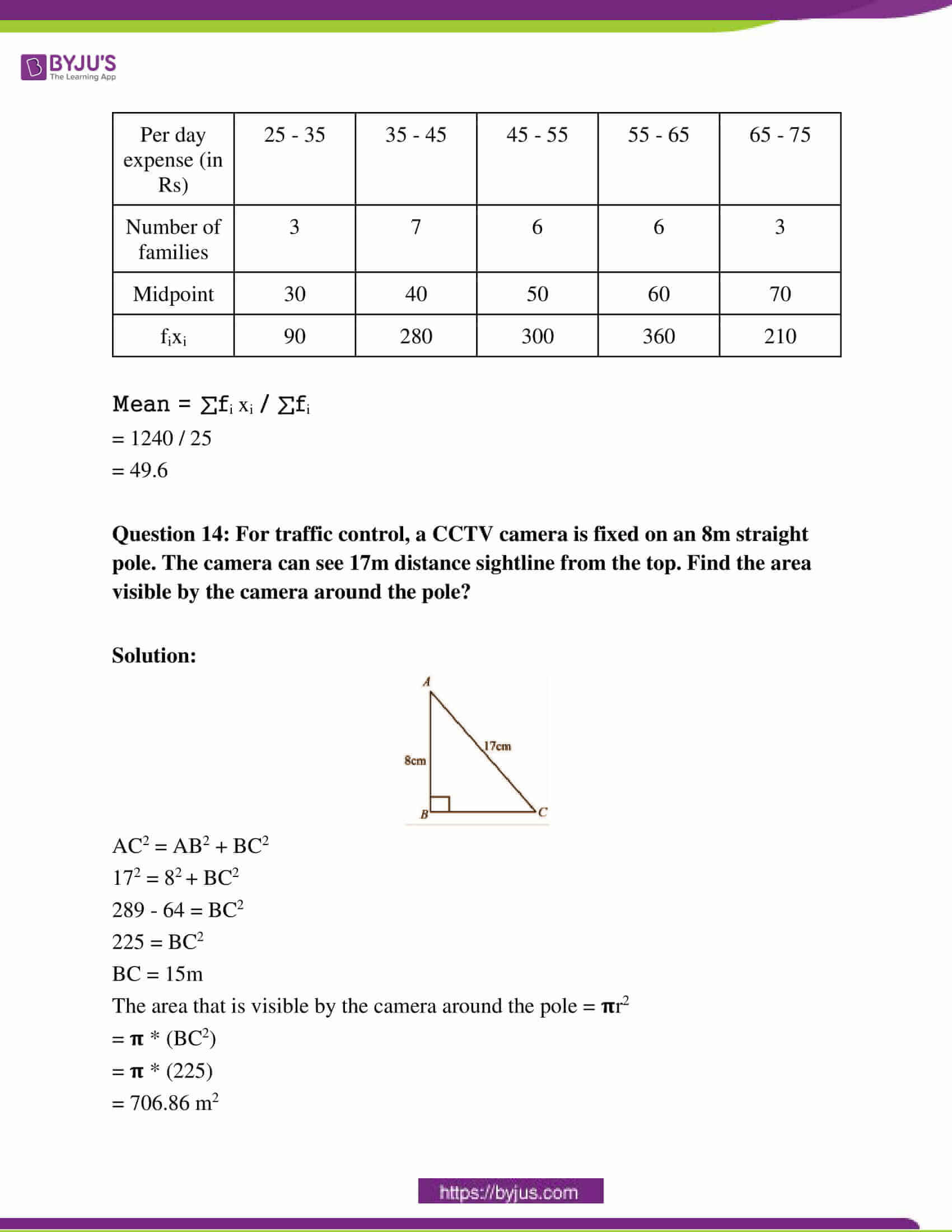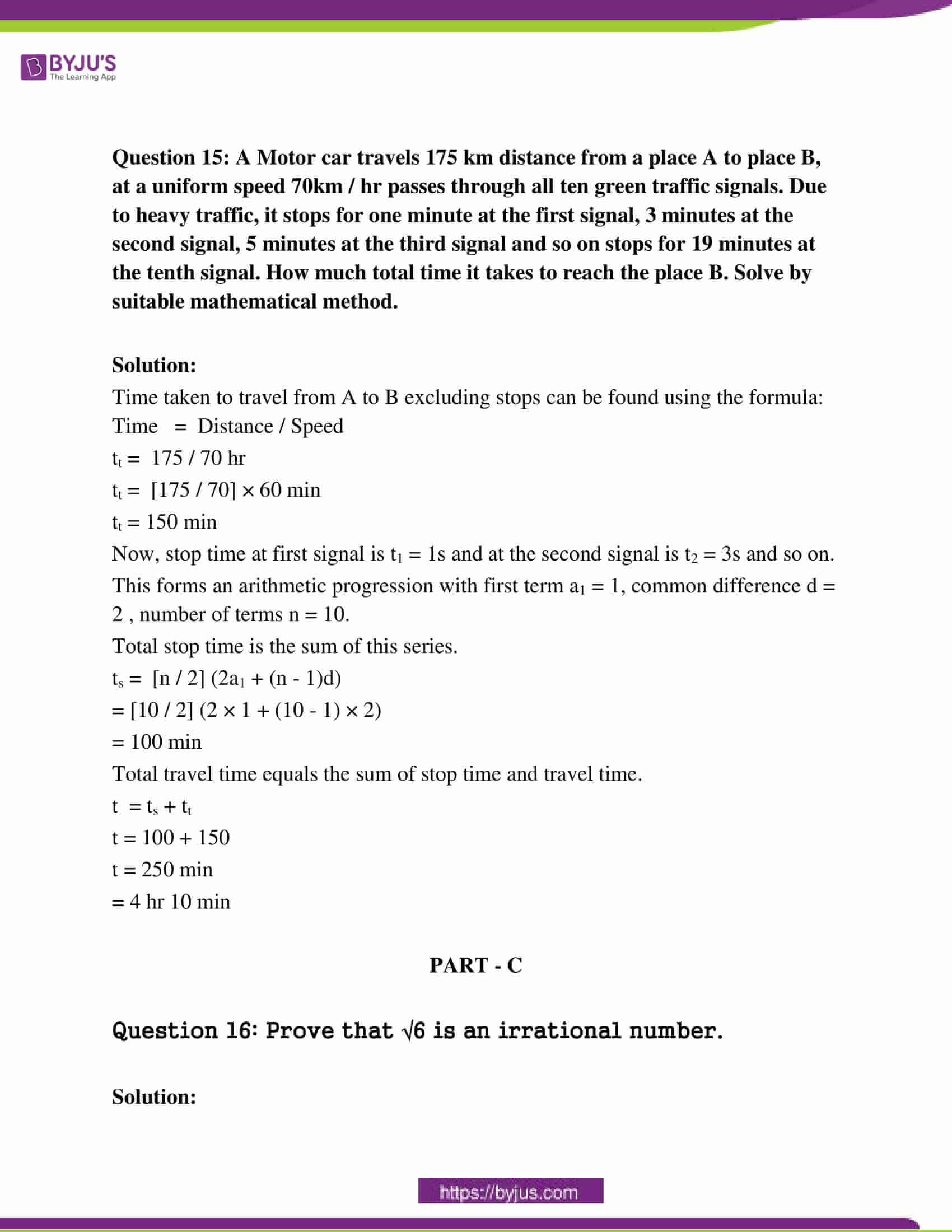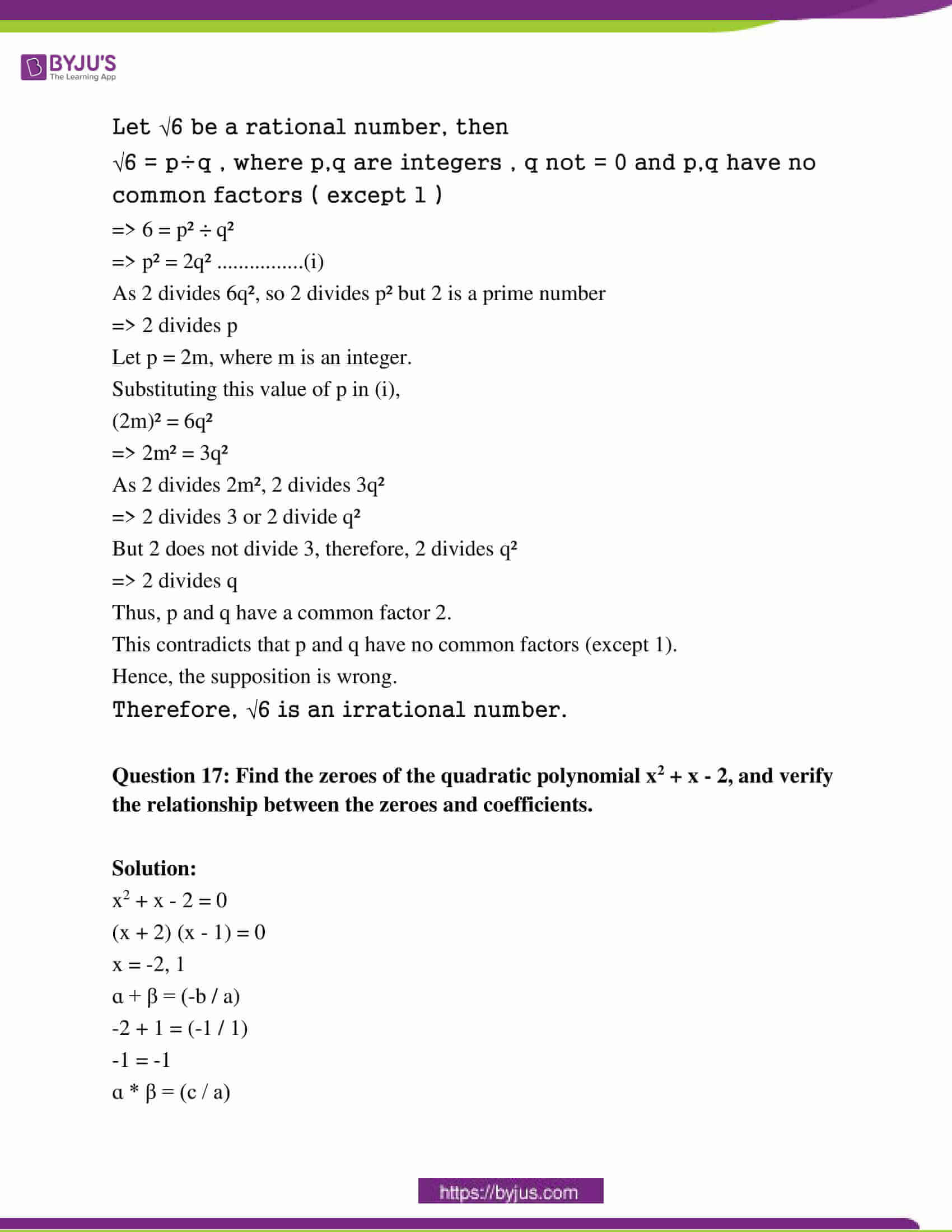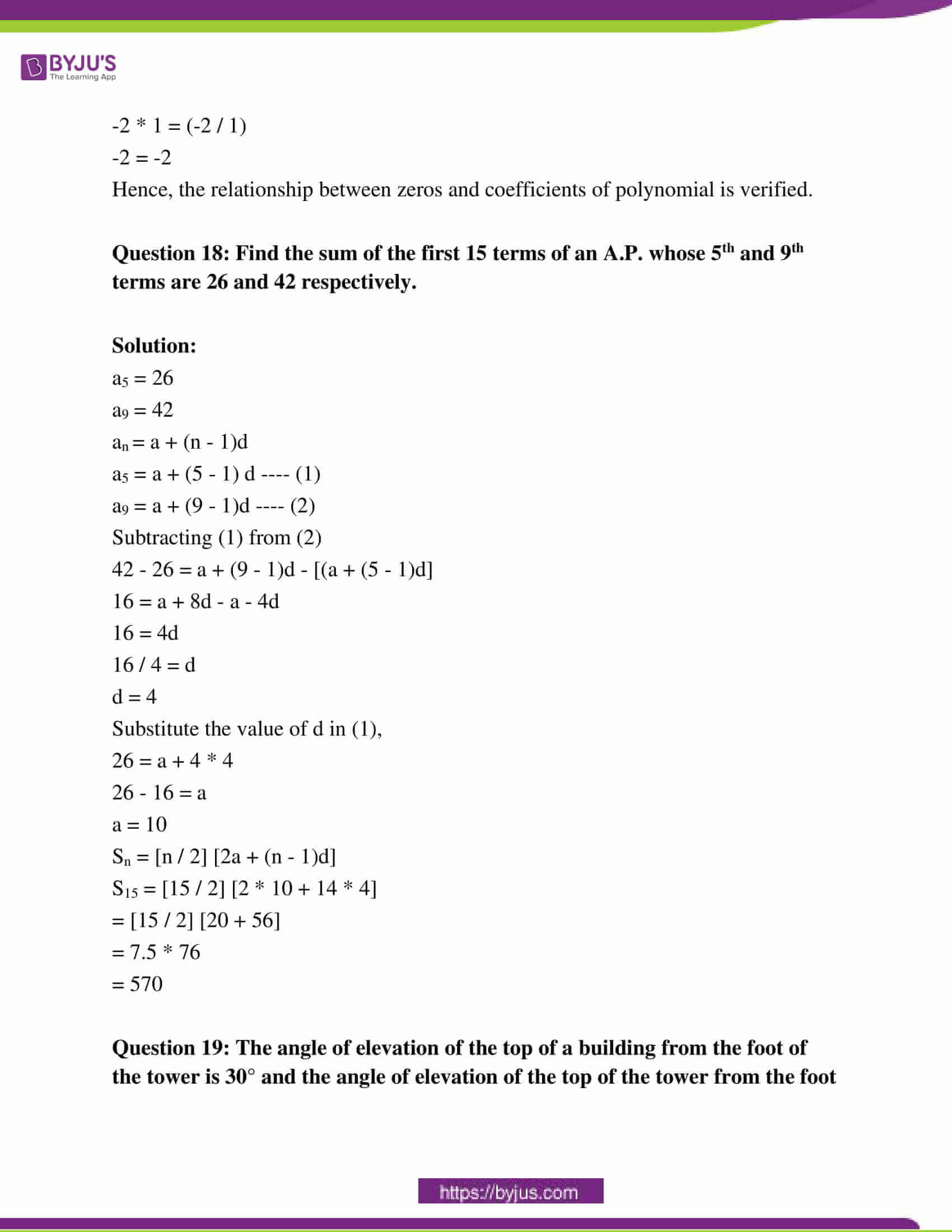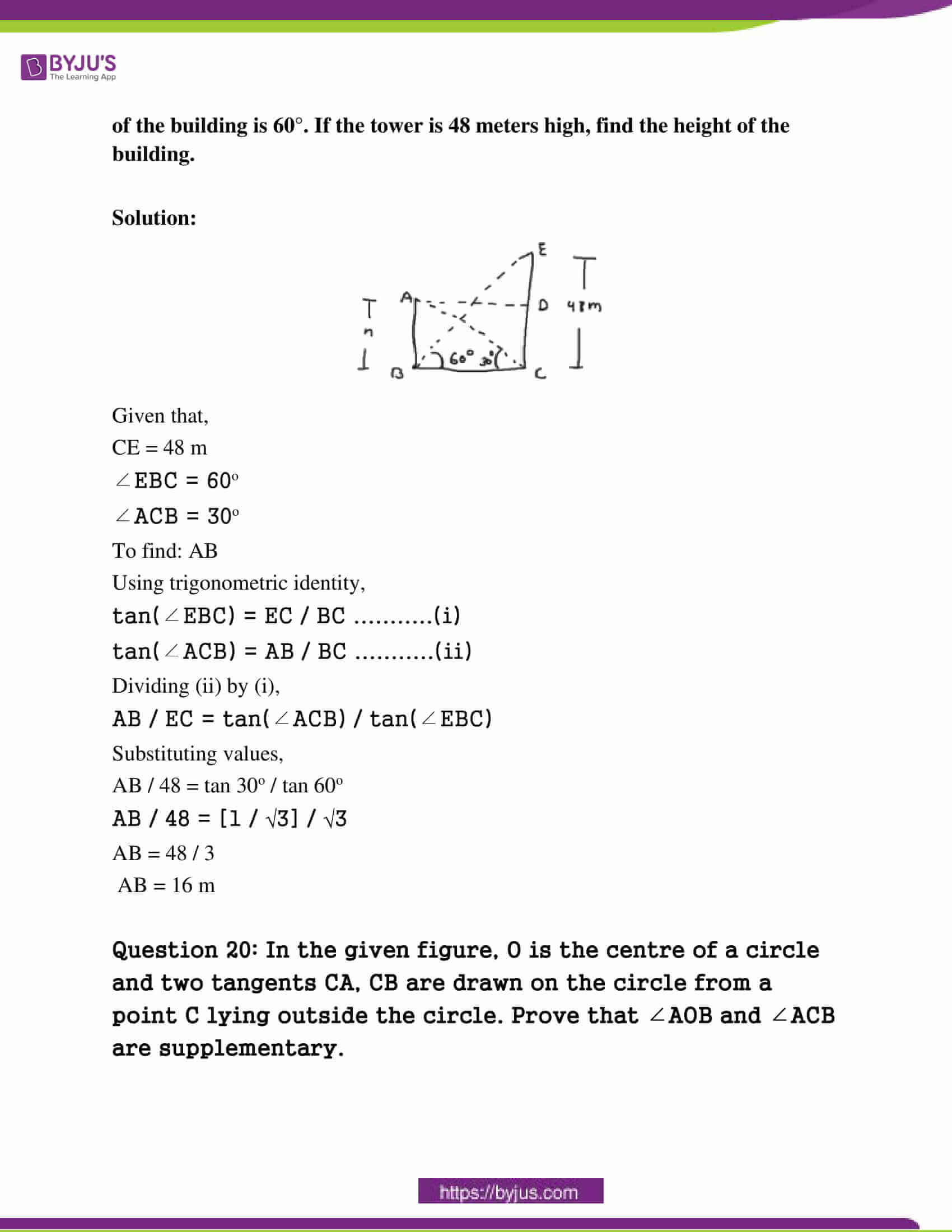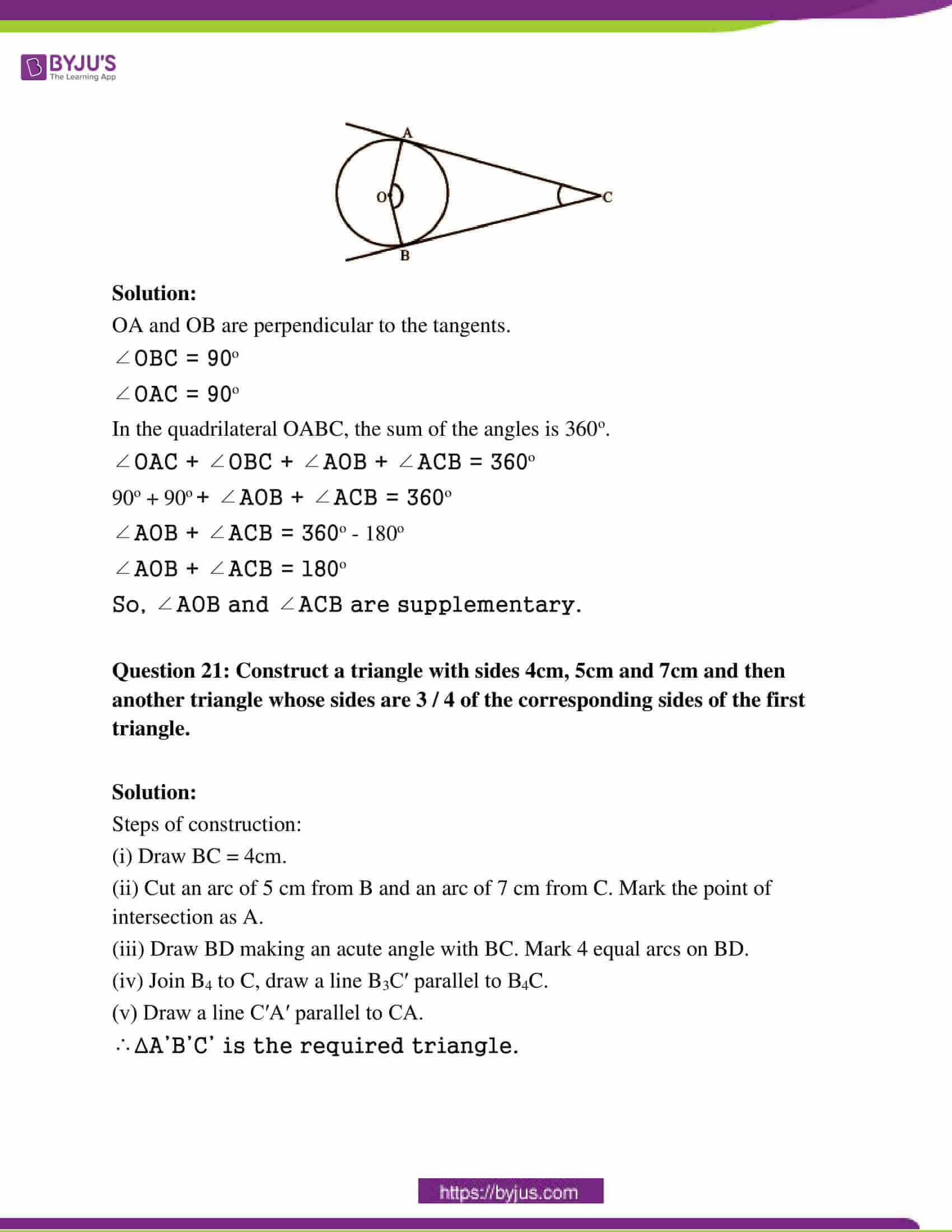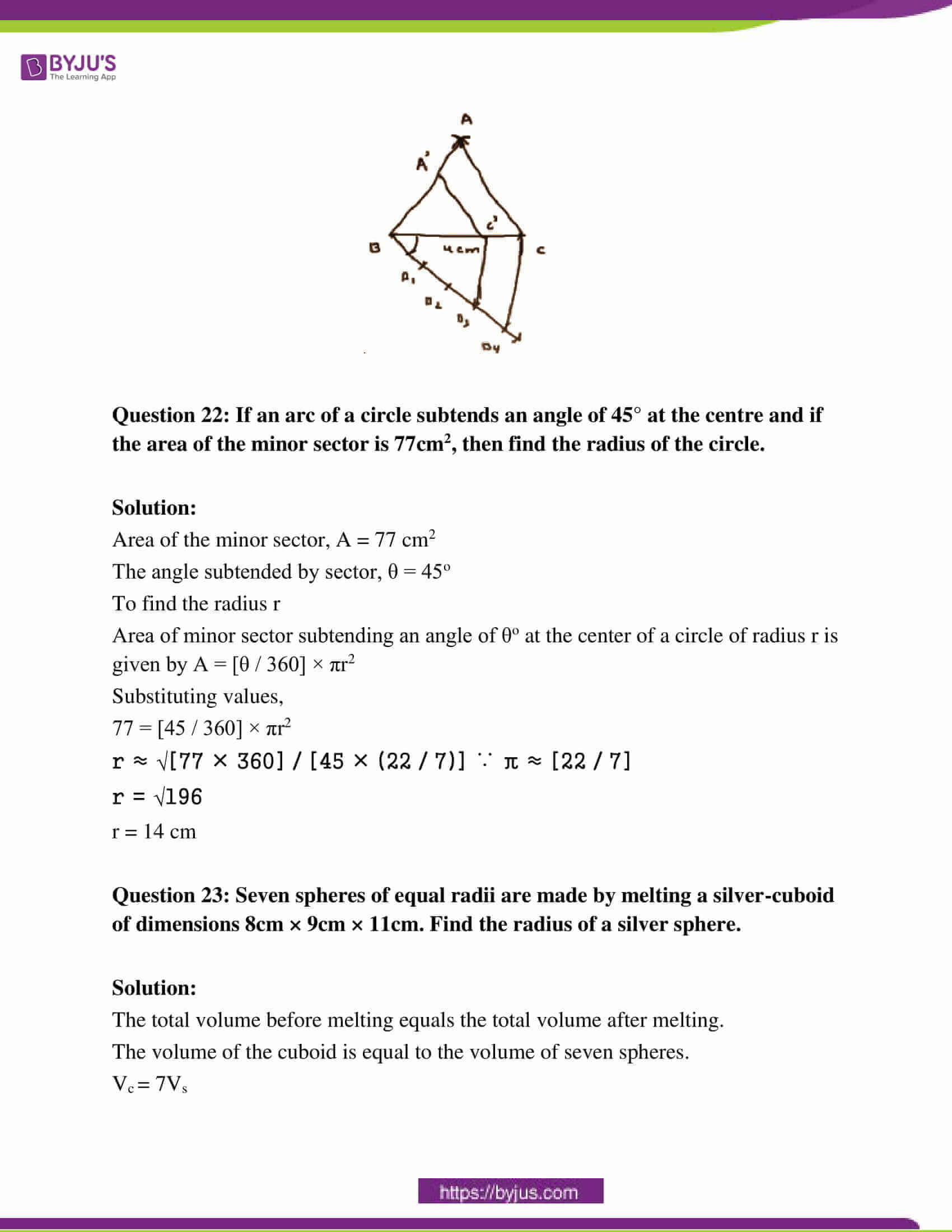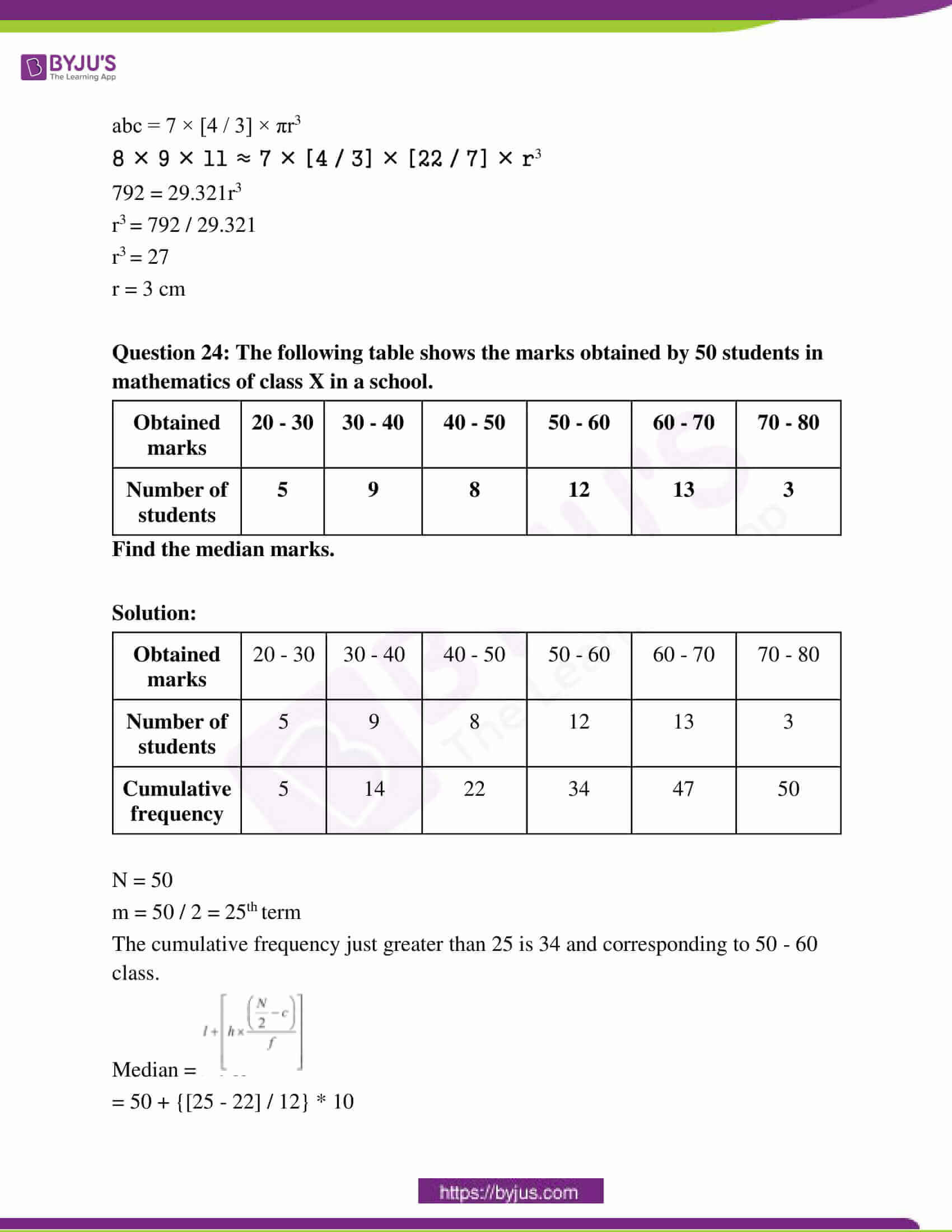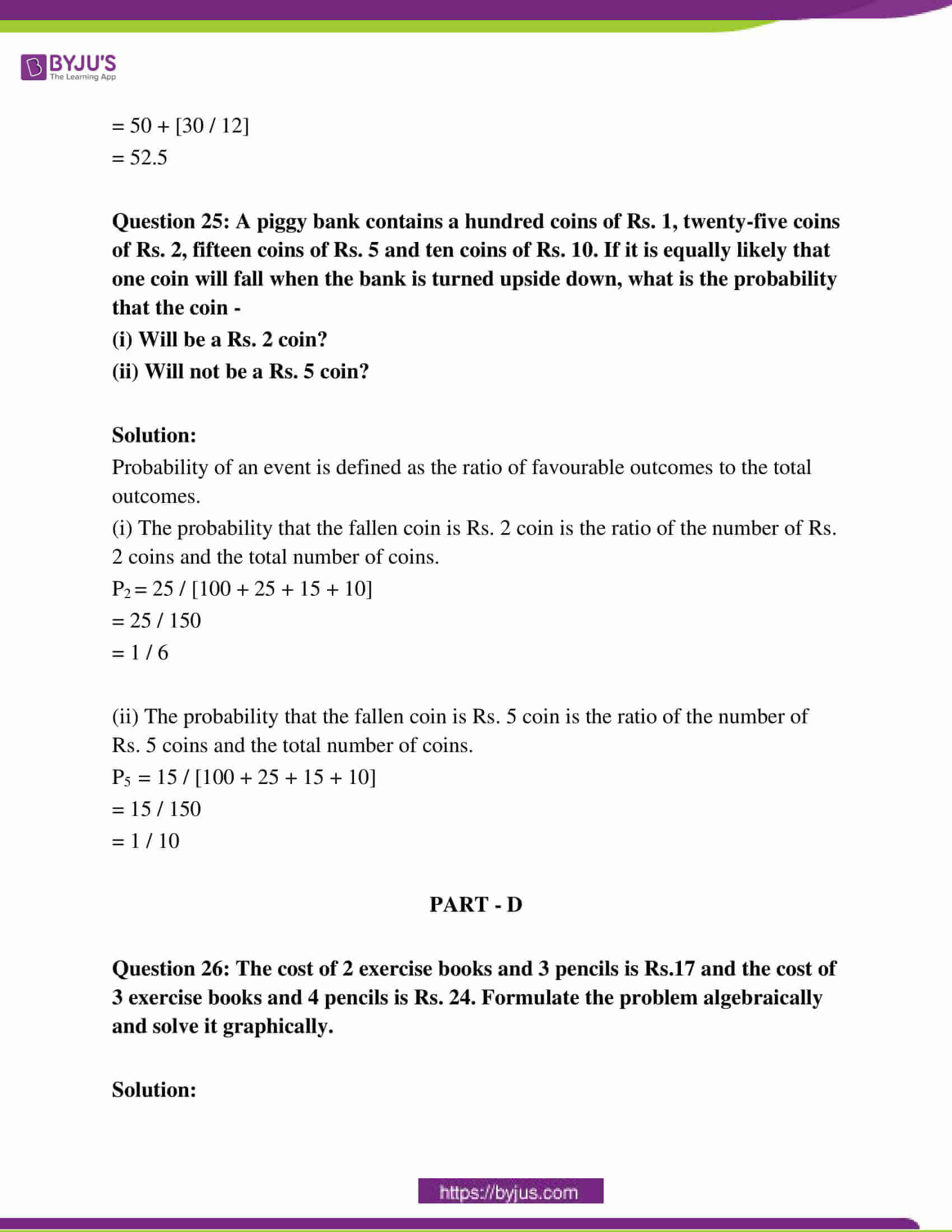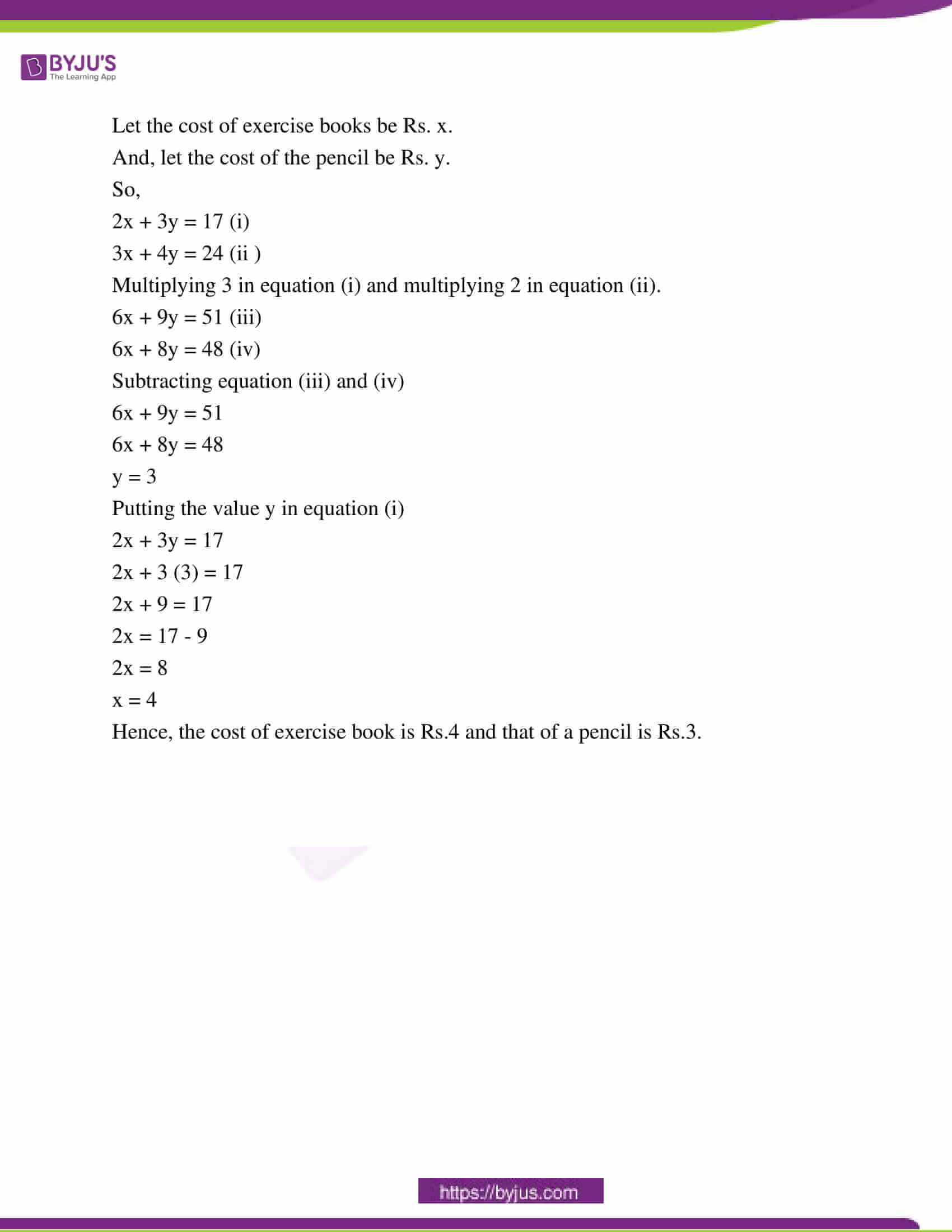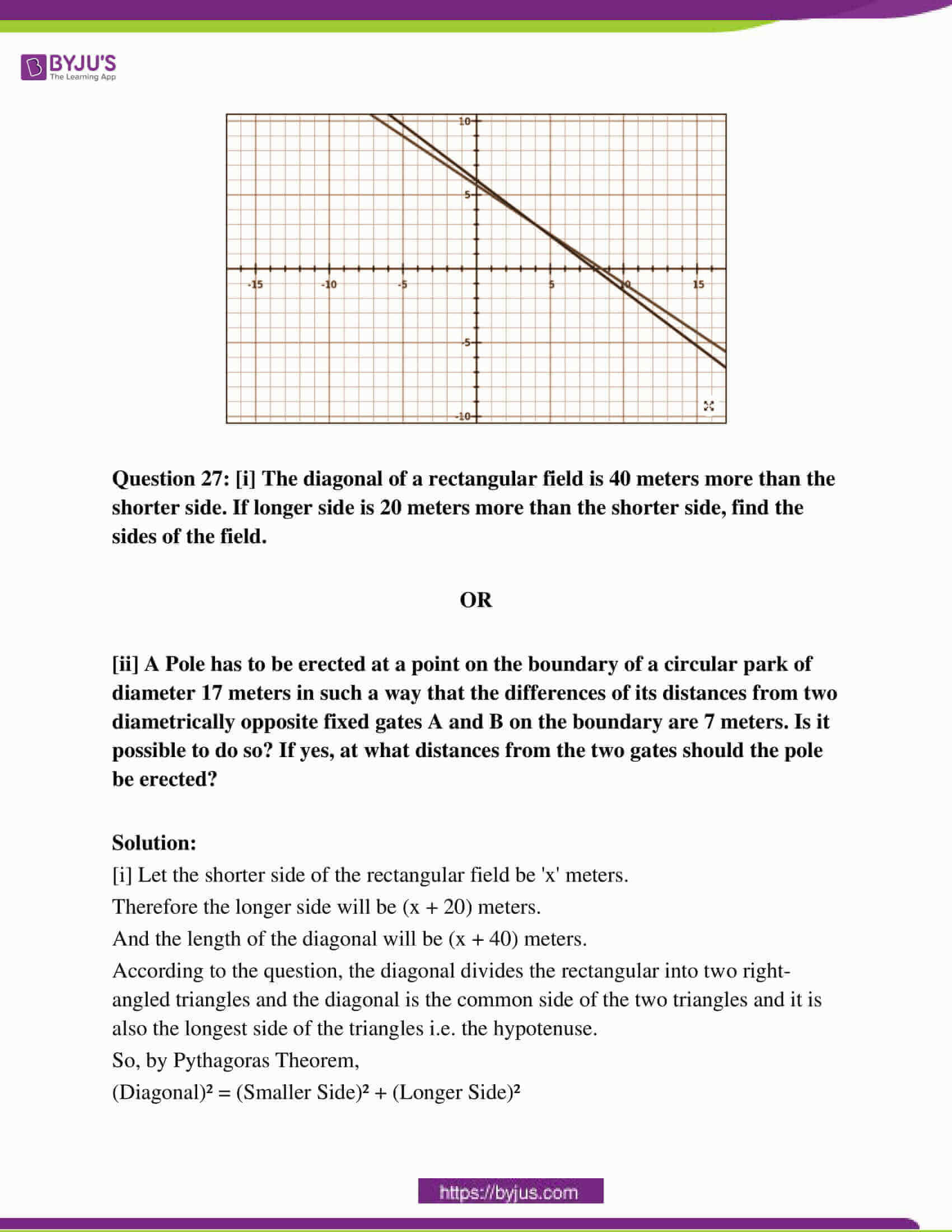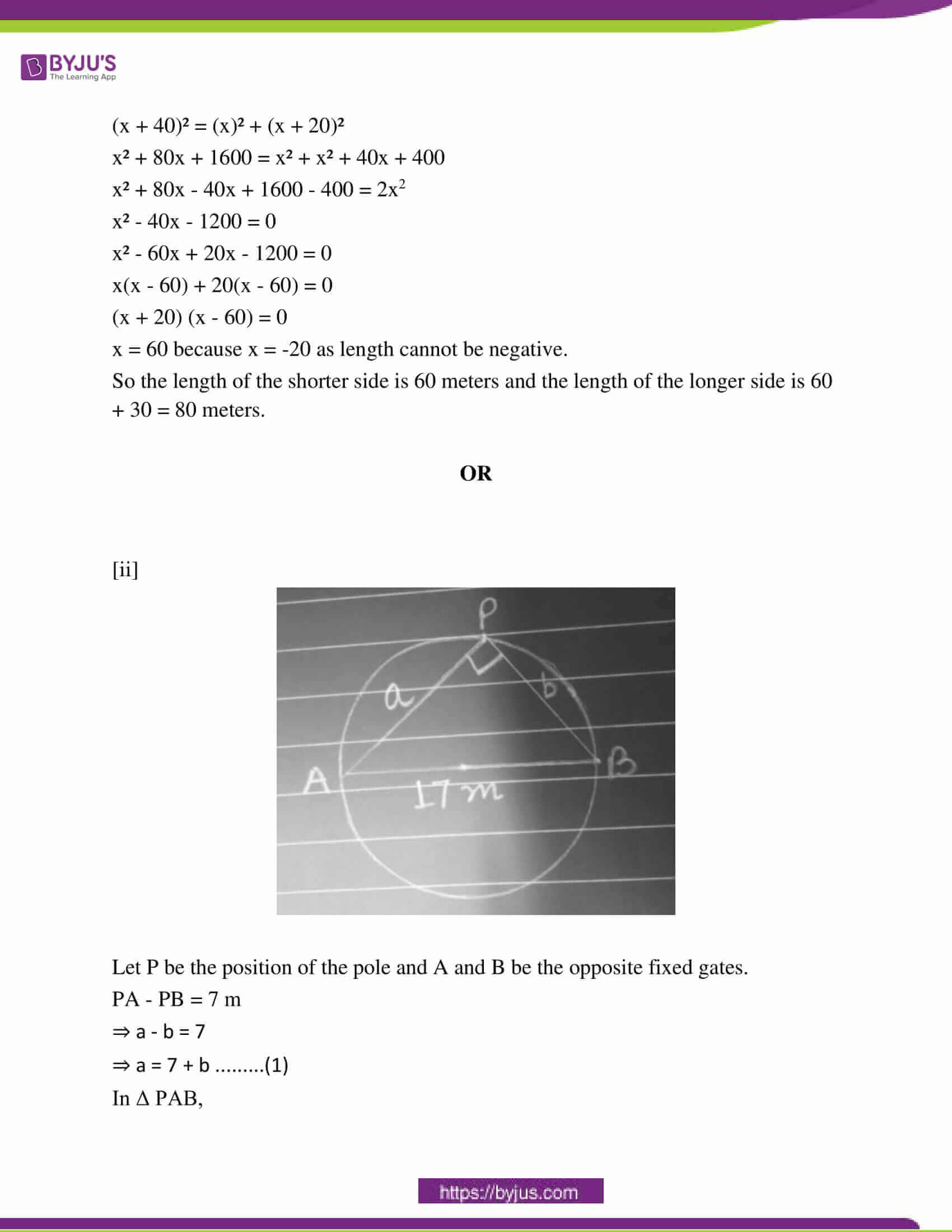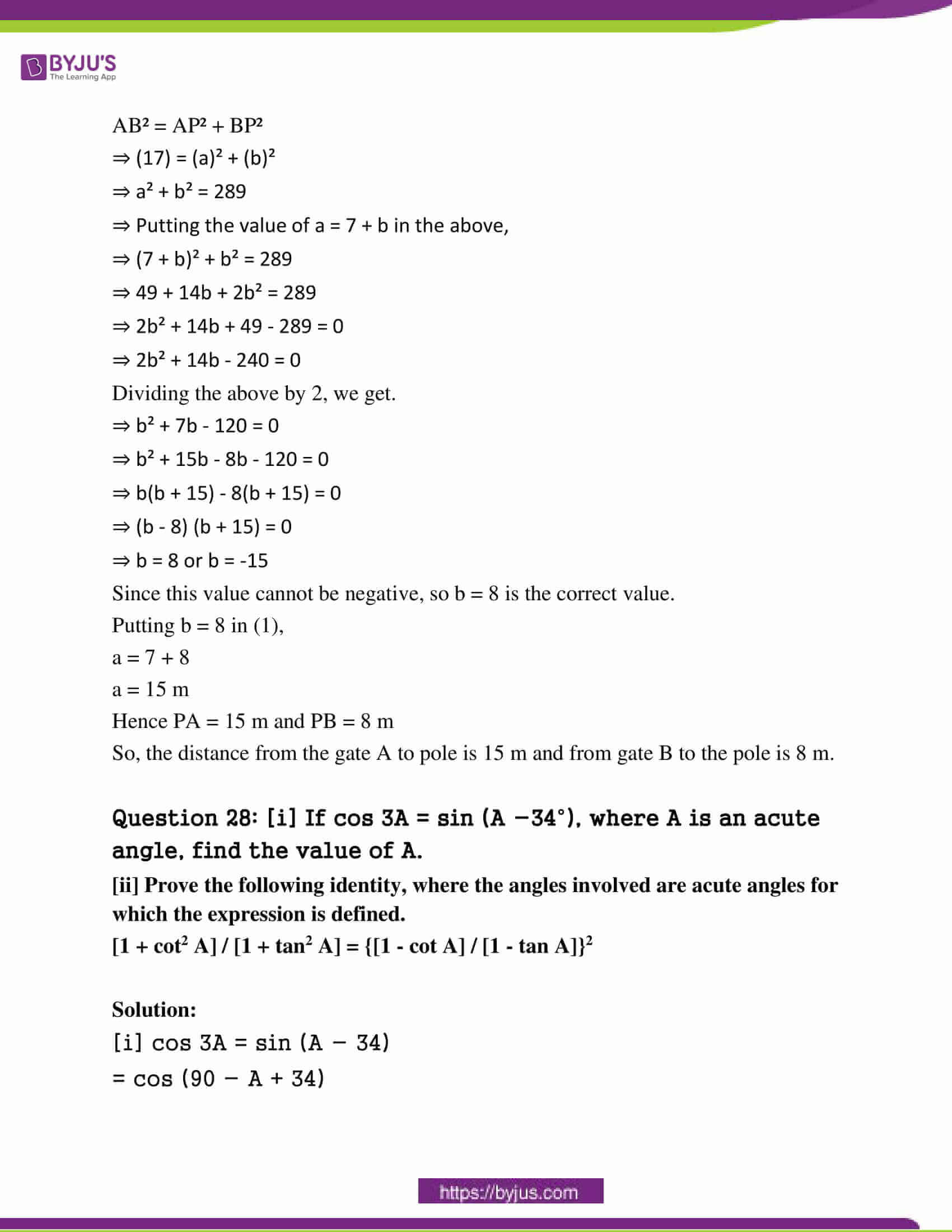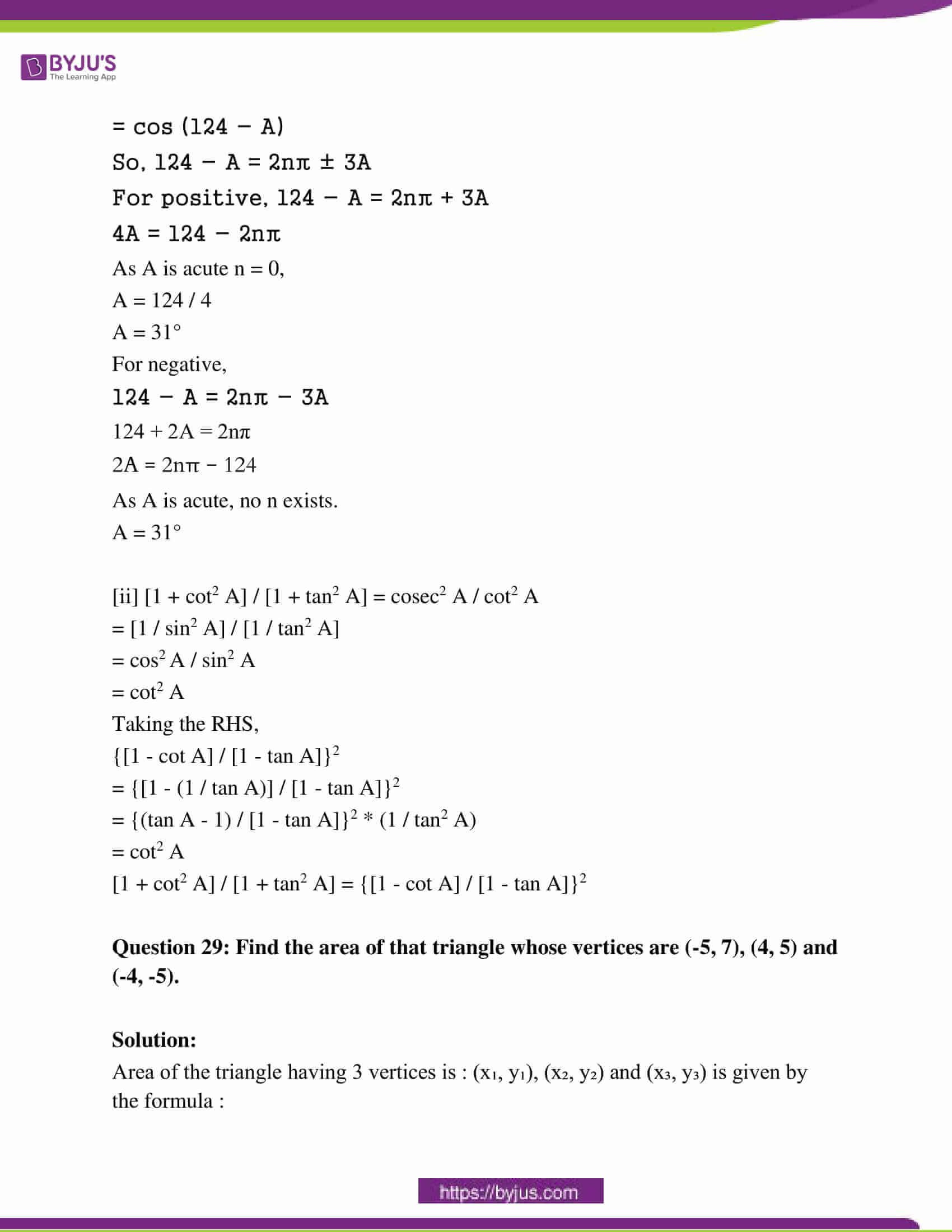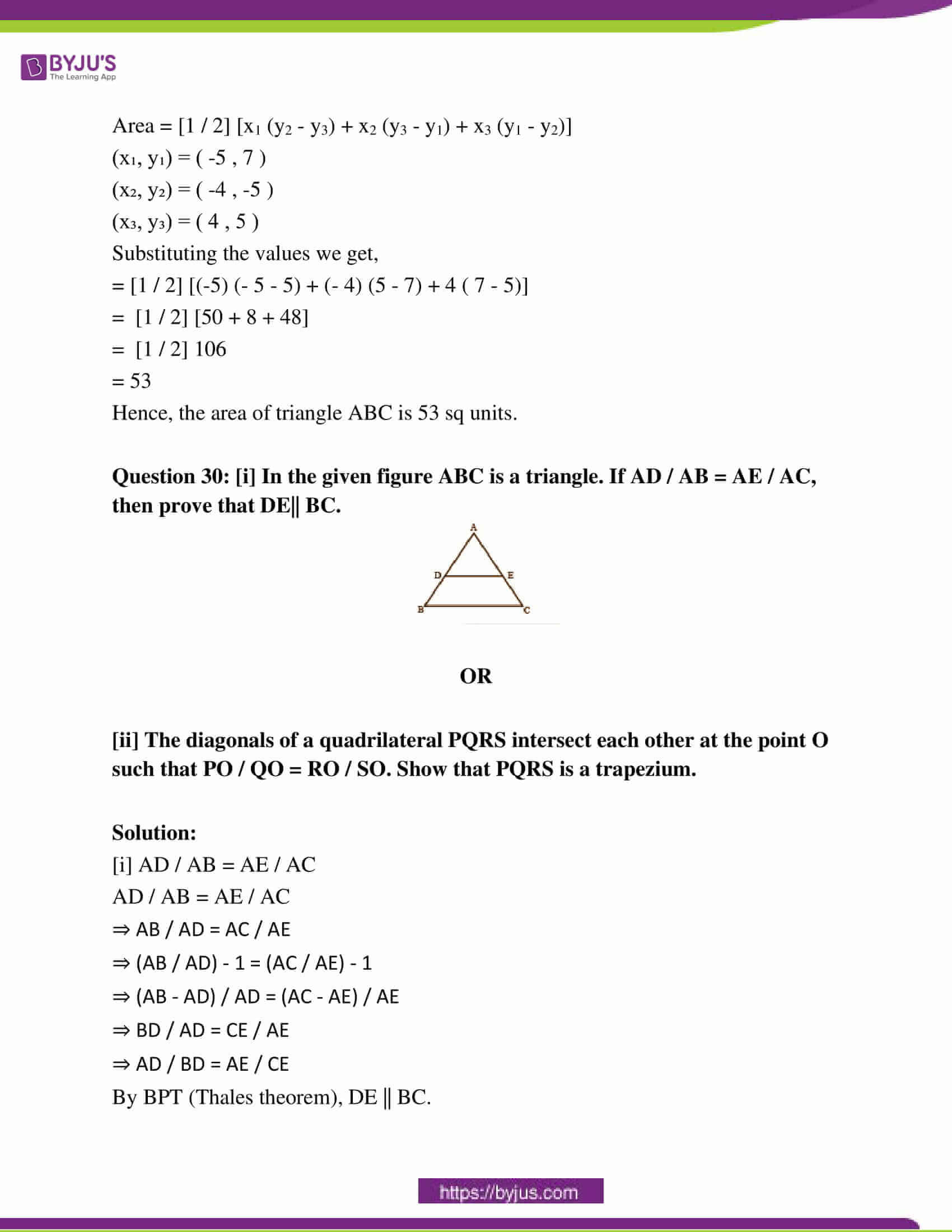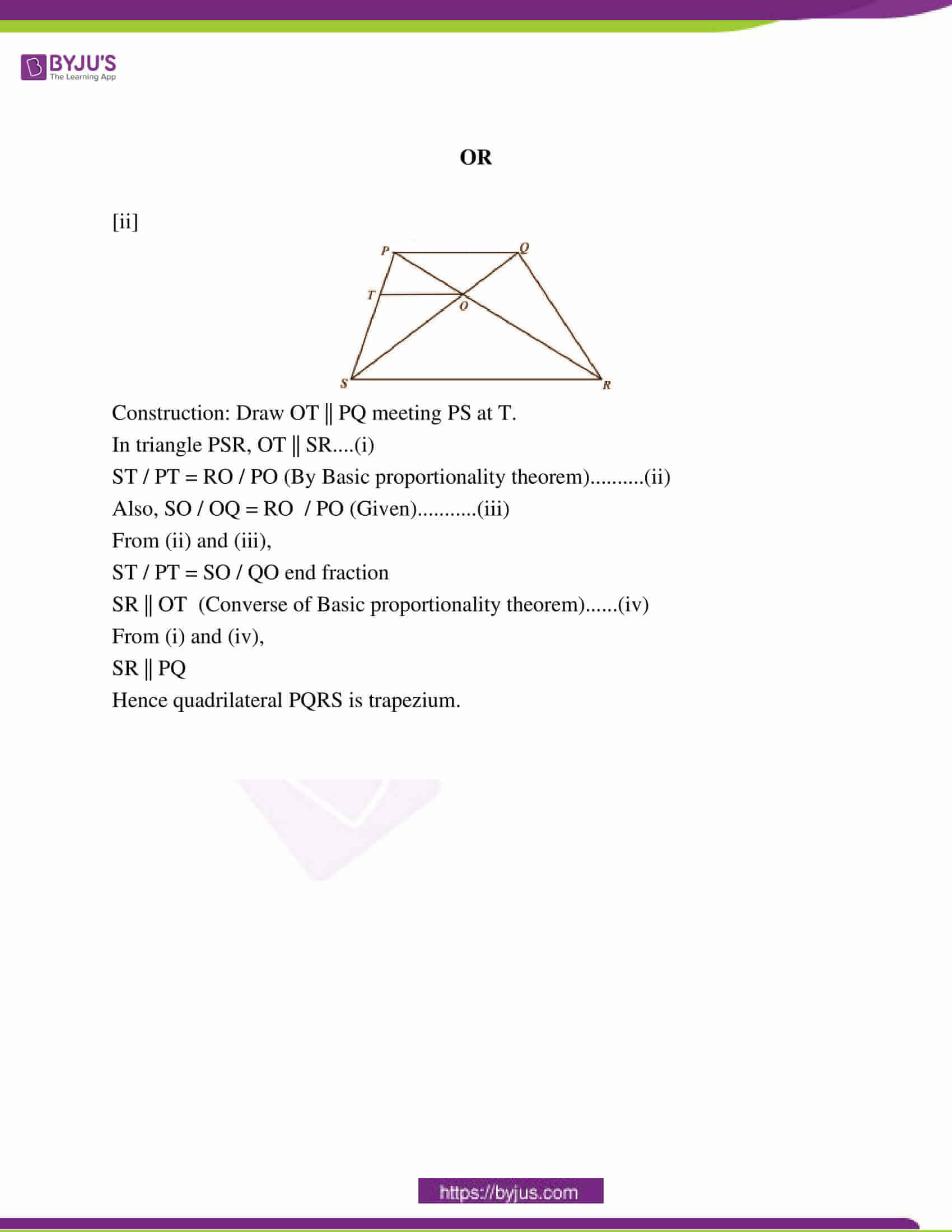### RBSE Class 10th Maths Question Paper With Solution 2016

PART – A

Question 1: Find the HCF of integers 375 and 675 by the prime factorisation method.

Solution:

375 = 53 × 3

675 = 52 × 33

HCF of the two numbers is x = 5a × 3b where a and b are the smallest of the integers occurring in the prime factorization of each number.

Hence, x = 52 × 31 = 75

Question 2: Find 11th term of the A.P. -17, -12, -7 …………………

Solution:

AP: -17, -12, -7 …………………

a = -17

d = -12 + 17 = 5

a11 = a + (n – 1) d

= -17 + (11 – 1) * 5

= -17 + 55 – 5

= 55 – 22

= 33

Question 3: If cos A = 12 / 13, then calculate cot A.

Solution:

cos A = 12 / 13

Base / hypotenuse = 12 / 13

Base = 12 units, hypotenuse = 13 units

(perpendicular)² = (hypotenuse)2 – (base)2

= 13² – 12²

=169 – 144

= 25

Perpendicular = √25 = 5 units

cot A = base / perpendicular

= 12 / 5

Question 4: Express the trigonometric ratio tan A in terms of sec A.

Solution:

tan2 A = sec2 A – 1

tan A = √sec2 A – 1

Question 5: The area of two similar triangles is in ratio 16:81. Find the ratio of its sides.

Solution:

The ratio of areas of two triangles is equal to the square of the ratio of sides of the triangles.

A1 / A2 = (S1 / S2)2

16 / 81 = (S1 / S2)2

√16 / 81 = (S1 / S2)

4 / 9 = (S1 / S2)

Question 6: In the given figure, O is the centre of a circle and two tangents QP and QR are drawn on the circle from a point Q lying outside the circle. Find the value of angle POR.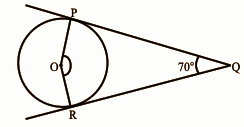Solution:

∠OPQ = 90o

∠ORQ = 90o

The sum of the angles of the quadrilateral OPQR is 360o.

∠OPQ + ∠ORQ + ∠PQR + ∠POR = 360o

90o + 90o + 70o + ∠POR = 360o

∠POR = 360o – 250o

∠POR = 110o

Question 7: How many tangents can be drawn on the circle of radius 5 cm from a point lying outside the circle at distance 9 cm from the centre?

Solution:

The number of tangents that can be drawn to a circle from a point lying outside the circle is two. Since the distance between the centre and the point (9 cm) is greater than the radius (5 cm), the point lies outside the circle.

Hence, two tangents can be drawn on the circle.

Question 8: Find the radius of that circle whose area is 616 cm2.

Solution:

The area of the circle is 616 cm2.

πr2 = 616

r = √616 / π

= √196

= 14 cm

Question 9: If the angle of the major sector of a circle is 250°. Then find the angle of the minor sector.

Solution:

The angle of the major sector is 250o.

Let the angle of the minor sector be x.

Circle = 360o

Circle = major sector + minor sector

360o = 250o + x

360o – 250o = x

x = 110o

Question 10: A coin is tossed once. Find the probability that it is not a tail.

Solution:

The total number of possible outcomes are head (H) and tail (T) = 2.

P (not getting a tail) = 1 / 2

PART – B

Question 11: If the middle point of two points A (-2, 5) and B (-5, y) is (-7 / 2, 3), then find the distance between points A and B.

Solution:

Let the middle point be P.

Using the distance formula,

AP = √([-7 / 2] – (-2))2 + (3 – 5)2

= √(9 / 4) + 4

= 5 / 2

AB = 2AP as P is the mid point of AB.

AB = 5

Question 12: The total surface area of a solid hemisphere is 462 cm2. Find its radius.

Solution:

Let r be the radius of the solid hemisphere.

The total surface area of solid hemisphere = 3πr2

⇒ 3πr2 = 462

⇒ πr2 = 154

⇒ r2 = 154 × [7 / 22]

⇒ r2 = 49

⇒ r = 7 cm

Question 13: Per day expenses of 25 families of the frequency distribution of a Dhani of a village is given as follows.

 Per day expense (in Rs) 25 – 35 35 – 45 45 – 55 55 – 65 65 – 75 Number of families 3 7 6 6 3

Find the mean expense of families by Direct Method.

Solution:

 Per day expense (in Rs) 25 – 35 35 – 45 45 – 55 55 – 65 65 – 75 Number of families 3 7 6 6 3 Midpoint 30 40 50 60 70 fixi 90 280 300 360 210

Mean = ∑fi xi / ∑fi

= 1240 / 25

= 49.6

Question 14: For traffic control, a CCTV camera is fixed on an 8m straight pole. The camera can see 17m distance sightline from the top. Find the area visible by the camera around the pole.

Solution: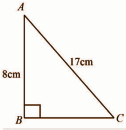AC2 = AB2 + BC2

172 = 82 + BC2

289 – 64 = BC2

225 = BC2

BC = 15m

The area that is visible by the camera around the pole = 𝛑r2

= 𝛑 * (BC2)

= 𝛑 * (225)

= 706.86 m2

Question 15: A Motor car travels 175 km distance from a place A to place B, at a uniform speed 70km / hr passes through all ten green traffic signals. Due to heavy traffic, it stops for one minute at the first signal, 3 minutes at the second signal, 5 minutes at the third signal and so on stops for 19 minutes at the tenth signal. How much total time it takes to reach the place B. Solve by suitable mathematical method.

Solution:

Time taken to travel from A to B excluding stops can be found using the formula: Time = Distance / Speed

tt = 175 / 70 hr

tt = [175 / 70] × 60 min

tt = 150 min

Now, the stop time at the first signal is t1 = 1s and at the second signal is t2 = 3s and so on.

This forms an arithmetic progression with first term a1 = 1, common difference d = 2 , number of terms n = 10.

Total stop time is the sum of this series.

ts = [n / 2] (2a1 + (n – 1)d)

= [10 / 2] (2 × 1 + (10 – 1) × 2)

= 100 min

Total travel time equals the sum of stop time and travel time.

t = ts + tt

t = 100 + 150

t = 250 min

= 4 hr 10 min

PART – C

Question 16: Prove that √6 is an irrational number.

Solution:

Let √6 be a rational number, then

√6 = p÷q , where p,q are integers , q not = 0 and p,q have no common factors ( except 1 )

=> 6 = p² ÷ q²

=> p² = 2q² …………….(i)

As 2 divides 6q², so 2 divides p² but 2 is a prime number

=> 2 divides p

Let p = 2m, where m is an integer.

Substituting this value of p in (i),

(2m)² = 6q²

=> 2m² = 3q²

As 2 divides 2m², 2 divides 3q²

=> 2 divides 3 or 2 divide q²

But 2 does not divide 3, therefore, 2 divides q²

=> 2 divides q

Thus, p and q have a common factor 2.

This contradicts that p and q have no common factors (except 1).

Hence, the supposition is wrong.

Therefore, √6 is an irrational number.

Question 17: Find the zeroes of the quadratic polynomial x2 + x – 2, and verify the relationship between the zeroes and coefficients.

Solution:

x2 + x – 2 = 0

(x + 2) (x – 1) = 0

x = -2, 1

ɑ + β = (-b / a)

-2 + 1 = (-1 / 1)

-1 = -1

ɑ * β = (c / a)

-2 * 1 = (-2 / 1)

-2 = -2

Hence, the relationship between zeros and coefficients of polynomials is verified.

Question 18: Find the sum of the first 15 terms of an A.P. whose 5th and 9th terms are 26 and 42, respectively.

Solution:

a5 = 26

a9 = 42

an = a + (n – 1)d

a5 = a + (5 – 1) d —- (1)

a9 = a + (9 – 1)d —- (2)

Subtracting (1) from (2)

42 – 26 = a + (9 – 1)d – [(a + (5 – 1)d]

16 = a + 8d – a – 4d

16 = 4d

16 / 4 = d

d = 4

Substitute the value of d in (1),

26 = a + 4 * 4

26 – 16 = a

a = 10

Sn = [n / 2] [2a + (n – 1)d]

S15 = [15 / 2] [2 * 10 + 14 * 4]

= [15 / 2] [20 + 56]

= 7.5 * 76

= 570

Question 19: The angle of elevation of the top of a building from the foot of the tower is 30° and the angle of elevation of the top of the tower from the foot of the building is 60°. If the tower is 48 meters high, find the height of the building.

Solution: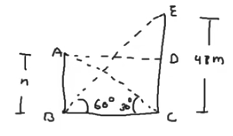Given that,

CE = 48 m

∠EBC = 60o

∠ACB = 30o

To find: AB

Using trigonometric identity,

tan(∠EBC) = EC / BC ………..(i)

tan(∠ACB) = AB / BC ………..(ii)

Dividing (ii) by (i),

AB / EC = tan(∠ACB) / tan(∠EBC)

​Substituting values,

AB / 48 = tan 30o / tan 60o

AB / 48 = [1 / √3] / √3

AB = 48 / 3

AB = 16 m

Question 20: In the given figure, O is the centre of a circle and two tangents CA, CB are drawn on the circle from a point C lying outside the circle. Prove that ∠AOB and ∠ACB are supplementary.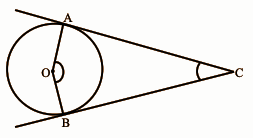Solution:

OA and OB are perpendicular to the tangents.

∠OBC = 90o

∠OAC = 90o

In the quadrilateral OABC, the sum of the angles is 360o.

∠OAC + ∠OBC + ∠AOB + ∠ACB = 360o

90o + 90o + ∠AOB + ∠ACB = 360o

∠AOB + ∠ACB = 360o – 180o

∠AOB + ∠ACB = 180o

So, ∠AOB and ∠ACB are supplementary.

Question 21: Construct a triangle with sides 4cm, 5cm and 7cm and then another triangle whose sides are 3 / 4 of the corresponding sides of the first triangle.

Solution:

Steps of construction:

(i) Draw BC = 4cm.

(ii) Cut an arc of 5 cm from B and an arc of 7 cm from C. Mark the point of intersection as A.

(iii) Draw BD making an acute angle with BC. Mark 4 equal arcs on BD.

(iv) Join B4 to C, draw a line B3C′ parallel to B4C.

(v) Draw a line C′A′ parallel to CA.

∴ΔA’B’C’ is the required triangle.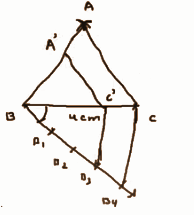Question 22: If an arc of a circle subtends an angle of 45° at the centre and if the area of the minor sector is 77cm2, then find the radius of the circle.

Solution:

Area of the minor sector, A = 77 cm2

The angle subtended by sector, θ = 45o

Area of minor sector subtending an angle of θo at the center of a circle of radius r is given by A = [θ / 360] × πr2

Substituting values,

77 = [45 / 360] × πr2

r ≈ √[77 × 360] / [45 × (22 / 7)] ∵ π ≈ [22 / 7]

r = √196

r = 14 cm

Question 23: Seven spheres of equal radii are made by melting a silver-cuboid of dimensions 8cm × 9cm × 11cm. Find the radius of a silver sphere.

Solution:

The total volume before melting equals the total volume after melting.

The volume of the cuboid is equal to the volume of seven spheres.

Vc = 7Vs

​abc = 7 × [4 / 3] × πr3

8 × 9 × 11 ≈ 7 × [4 / 3] × [22 / 7] × r3

792 = 29.321r3

r3 = 792 / 29.321

r3 = 27

r = 3 cm

Question 24: The following table shows the marks obtained by 50 students in mathematics of class X in a school.

 Obtained marks 20 – 30 30 – 40 40 – 50 50 – 60 60 – 70 70 – 80 Number of students 5 9 8 12 13 3

Find the median marks.

Solution:

 Obtained marks 20 – 30 30 – 40 40 – 50 50 – 60 60 – 70 70 – 80 Number of students 5 9 8 12 13 3 Cumulative frequency 5 14 22 34 47 50

N = 50

m = 50 / 2 = 25th term

The cumulative frequency just greater than 25 is 34 and corresponds to 50 – 60 class.

Median =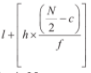= 50 + {[25 – 22] / 12} * 10

= 50 + [30 / 12]

= 52.5

Question 25: A piggy bank contains a hundred coins of Rs. 1, twenty-five coins of Rs. 2, fifteen coins of Rs. 5 and ten coins of Rs. 10. If it is equally likely that one coin will fall when the bank is turned upside down, what is the probability that the coin –

(i) Will it be a Rs. 2 coin?

(ii) Will not be a Rs. 5 coin?

Solution:

Probability of an event is defined as the ratio of favourable outcomes to the total outcomes.

(i) The probability that the fallen coin is Rs. 2 coin is the ratio of the number of Rs. 2 coins and the total number of coins.

P2 = 25 / [100 + 25 + 15 + 10]

= 25 / 150

= 1 / 6

(ii) The probability that the fallen coin is Rs. 5 coin is the ratio of the number of Rs. 5 coins and the total number of coins.

P5 = 15 / [100 + 25 + 15 + 10]

= 15 / 150

= 1 / 10

PART – D

Question 26: The cost of 2 exercise books and 3 pencils is Rs.17 and the cost of 3 exercise books and 4 pencils is Rs. 24. Formulate the problem algebraically and solve it graphically.

Solution:

Let the cost of exercise books be Rs. x.

And, let the cost of the pencil be Rs. y.

So,

2x + 3y = 17 (i)

3x + 4y = 24 (ii )

Multiplying 3 in equation (i) and multiplying 2 in equation (ii).

6x + 9y = 51 (iii)

6x + 8y = 48 (iv)

Subtracting equation (iii) and (iv)

6x + 9y = 51

6x + 8y = 48

y = 3

Putting the value y in equation (i)

2x + 3y = 17

2x + 3 (3) = 17

2x + 9 = 17

2x = 17 – 9

2x = 8

x = 4

Hence, the cost of exercise book is Rs.4 and that of a pencil is Rs.3.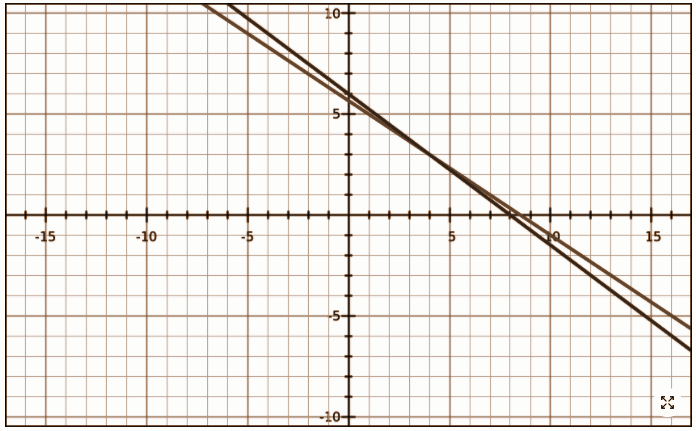Question 27: [i] The diagonal of a rectangular field is 40 meters more than the shorter side. If the longer side is 20 meters more than the shorter side, find the sides of the field.

OR

[ii] A Pole has to be erected at a point on the boundary of a circular park of diameter 17 meters in such a way that the differences of its distances from two diametrically opposite fixed gates A and B on the boundary are 7 meters. Is it possible to do so? If yes, at what distances from the two gates should the pole be erected?

Solution:

[i] Let the shorter side of the rectangular field be ‘x’ meters.

Therefore the longer side will be (x + 20) meters.

And the length of the diagonal will be (x + 40) meters.

According to the question, the diagonal divides the rectangular into two right-angled triangles and the diagonal is the common side of the two triangles and it is also the longest side of the triangles i.e. the hypotenuse.

So, by Pythagoras Theorem,

(Diagonal)² = (Smaller Side)² + (Longer Side)²

(x + 40)² = (x)² + (x + 20)²

x² + 80x + 1600 = x² + x² + 40x + 400

x² + 80x – 40x + 1600 – 400 = 2x2

x² – 40x – 1200 = 0

x² – 60x + 20x – 1200 = 0

x(x – 60) + 20(x – 60) = 0

(x + 20) (x – 60) = 0

x = 60 because x = -20 as length cannot be negative.

So the length of the shorter side is 60 meters and the length of the longer side is 60 + 30 = 80 meters.

OR

[ii]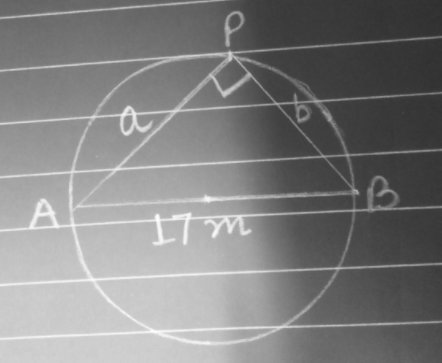Let P be the position of the pole and A and B be the opposite fixed gates.

PA – PB = 7 m

⇒ a – b = 7

⇒ a = 7 + b ………(1)

In Δ PAB,

AB² = AP² + BP²

⇒ (17) = (a)² + (b)²

⇒ a² + b² = 289

⇒ Putting the value of a = 7 + b in the above,

⇒ (7 + b)² + b² = 289

⇒ 49 + 14b + 2b² = 289

⇒ 2b² + 14b + 49 – 289 = 0

⇒ 2b² + 14b – 240 = 0

Dividing the above by 2, we get.

⇒ b² + 7b – 120 = 0

⇒ b² + 15b – 8b – 120 = 0

⇒ b(b + 15) – 8(b + 15) = 0

⇒ (b – 8) (b + 15) = 0

⇒ b = 8 or b = -15

Since this value cannot be negative, so b = 8 is the correct value.

Putting b = 8 in (1),

a = 7 + 8

a = 15 m

Hence PA = 15 m and PB = 8 m

So, the distance from gate A to pole is 15 m and from gate B to the pole is 8 m.

Question 28: [i] If cos 3A = sin (A −34°), where A is an acute angle, find the value of A.

[ii] Prove the following identity, where the angles involved are acute angles for which the expression is defined.

[1 + cot2 A] / [1 + tan2 A] = {[1 – cot A] / [1 – tan A]}2

Solution:

[i] cos 3A = sin (A − 34)

= cos (90 − A + 34)

= cos (124 − A)

So, 124 − A = 2nπ ± 3A

For positive, 124 − A = 2nπ + 3A

4A = 124 − 2nπ

As A is acute n = 0,

A = 124 / 4

A = 31°

For negative,

124 − A = 2nπ − 3A

124 + 2A = 2nπ

2A = 2nπ − 124

As A is acute, no n exists.

A = 31°

[ii] [1 + cot2 A] / [1 + tan2 A] = cosec2 A / cot2 A

= [1 / sin2 A] / [1 / tan2 A]

= cos2 A / sin2 A

= cot2 A

Taking the RHS,

{[1 – cot A] / [1 – tan A]}2

= {[1 – (1 / tan A)] / [1 – tan A]}2

= {(tan A – 1) / [1 – tan A]}2 * (1 / tan2 A)

= cot2 A

[1 + cot2 A] / [1 + tan2 A] = {[1 – cot A] / [1 – tan A]}2

Question 29: Find the area of that triangle whose vertices are (-5, 7), (4, 5) and (-4, -5).

Solution:

Area of the triangle having 3 vertices is : (x₁, y₁), (x₂, y₂) and (x₃, y₃) is given by the formula :

Area = [1 / 2] [x1 (y2 – y3) + x2 (y3 – y1) + x3 (y1 – y2)]

(x₁, y₁) = ( -5 , 7 )

(x₂, y₂) = ( -4 , -5 )

(x₃, y₃) = ( 4 , 5 )

Substituting the values we get,

= [1 / 2] [(-5) (- 5 – 5) + (- 4) (5 – 7) + 4 ( 7 – 5)]

= [1 / 2] [50 + 8 + 48]

= [1 / 2] 106

= 53

Hence, the area of triangle ABC is 53 sq units.

Question 30: [i] In the given figure ABC is a triangle. If AD / AB = AE / AC, then prove that DE|| BC.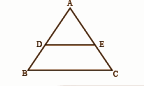OR

[ii] The diagonals of a quadrilateral PQRS intersect each other at the point O such that PO / QO = RO / SO. Show that PQRS is a trapezium.

Solution:

[i] AD / AB = AE / AC

AD / AB = AE / AC

⇒ AB / AD = AC / AE

⇒ (AB / AD) – 1 = (AC / AE) – 1

⇒ (AB – AD) / AD = (AC – AE) / AE

⇒ BD / AD = CE / AE

⇒ AD / BD = AE / CE

By BPT (Thales theorem), DE || BC.

OR

[ii]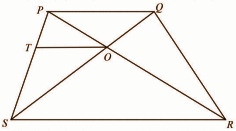Construction: Draw OT || PQ meeting PS at T.

In triangle PSR, OT || SR….(i)

ST / PT = RO / PO (By Basic proportionality theorem)……….(ii)

Also, SO / OQ = RO / PO (Given)………..(iii)

From (ii) and (iii),

ST / PT = SO / QO end fraction

SR || OT (Converse of Basic proportionality theorem)……(iv)

From (i) and (iv),

SR || PQ